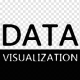R 做為一個專為統計與圖表打造的軟體來說，相當適合做資料分析與處理；比方說，若我們要拆解資料的主要特徵，PCA ( Principal Component Analysis ) 是個不錯的演算法，而相較於一般需要自行撰寫該演算法的程式語言來說，「princomp」這個計算 PCA 的函式便已內建在 R 的環境之中。除了統計的應用外，也有像是 ggplot2 這類的強大圖表函式庫；下圖即為由 R Studio 製作的 ggplot2 指令速查表：( ggplot2 cheat sheet by R Studio, CC-BY 4.0. source:  http://www.rstudio.com/wp-content/uploads/2015/12/ggplot2-cheatsheet-2.0.pdf)

## 什麼是 Shiny？

• ui.R — 前端程式碼，描述我們的專案網頁要怎樣呈現與排版
• server.R — 後端程式碼，負責分析、計算與繪製圖表，並將結果傳遞給前端。

Shiny 並不負責圖表的計算，但他為我們隱藏了網頁互動與設計的細節，並內建了各種表單元件，讓我們可以直接透過 R 的語法來設置元件並利用來達成互動效果。

### 基本建置

```  install.packages("shiny")
library(shiny)```

`  runExample("01_hello")`server.R

`  shinyServer(function(input, output) { })`

ui.R

```  library(shiny)
shinyUI(fluidPage())```

### ui.R — 視覺化前端

ui.R 這隻檔案負責告訴 Shiny 我們的網頁前端要長什麼樣子。一般來說，設計網頁我們會利用 HTML 、 CSS 等技術來實現，但為了讓設計的門檻再降低，Shiny 預先包裝好了許多實用的表單與排版元素，讓我們可以很輕易的在圖表中加入各種控制項。

```  library(shiny)
shinyUI(fluidPage(
))```

```  library(shiny)
shinyUI(fluidPage(
titlePanel("大家好")
))```

```  library(shiny)
shinyUI(fluidPage(
titlePanel("大家好"),
titlePanel("大家好"),
titlePanel("大家好")
))```• sidebarLayout — 將頁面切成兩塊，包含主內容與 sidebar
• sidebarPanel — 在 sidebar 內使用的面版
• mainPanel — 主要呈現資料的面版
• navlistPanel — 導覽面板
• tabPanel — 頁籤面板

```  radioButtons("radio", label = "Choices", choices = list("Choice 1" = 1, "Choice 2" = 2)),
sliderInput("slider1", label = "Slider", min = 0, max = 100, value = 50),``````  library(shiny)
shinyUI(fluidPage(
titlePanel("大家好"),
sidebarLayout(
sidebarPanel(
radioButtons("radio", label = "Choices", choices = list("Choice 1" = 1, "Choice 2" = 2)),
sliderInput("slider1", label = "Slider", min = 0, max = 100, value = 50)
),
mainPanel("逮給厚，哇喜逮頑歐郎")
)
))```

1. 使用 titlePanel 設定標題
2. sidebarLayout 將畫面左右切分
3. sidebarPanel 設定左方的面板，內含 Radio 與 Slider 控制項
4. mainPanel 裡面只寫上「逮給厚，哇喜逮頑歐郎」等字。Shiny 的控制項其實是基於另一個網路套件「 Bootstrap 」製作出來的，支援的元件數量不少，有興趣的讀者可以參考 Widget Gallery 這個網頁了解更多。裡面除了列出支援的套件，也提供使用的語法，下圖為其中一部份元件範例的截圖：### server.R — 計算與繪圖的後端

```  shinyServer(function(input, output) {
output\$distPlot <- renderPlot({
x <- faithful[, 2] # Old Faithful Geyser data
bins <- seq(min(x), max(x), length.out = 10)
hist(x, breaks = bins, col = 'blue', border = 'white')
})
})```

`  mainPanel(plotOutput("distPlot"))``  sliderInput("slider1", label = "Slider", min = 0, max = 100, value = 50)`

`  bins <- seq(min(x), max(x), length.out = input\$slider + 1)`## 小結

「你什麼都不用會，只要會 R ，就可以做互動圖表。」

• 需要更複雜客製化的時候， R 語言包裝的 HTML 會是場災難。
• 無法更即時的互動。圖片永遠由 server 端重新計算後產生。
• 視覺呈現受制於 R 套件，仍無法利用 D3.js 等更強大的視覺函式庫。yangyang

Bug在最後面的互動式圖表UI跟Server (如下的程式碼)

sliderInput(“slider1”, label = “Slider”, min = 0, max = 100, value = 50)
bins <- seq(min(x), max(x), length.out = input\$slider + 1)openopen114

bins <- seq(min(x), max(x), length.out = input\$slider + 1)